# Eddy Current Non-Destructive Characterization of Carbon Fiber Reinforcement Composites Considering Capacitive Effect

Eddy Current Non-Destructive Characterization of Carbon Fiber Reinforcement Composites Considering Capacitive Effect

Samir BensaidOmar Adib Safer Didier Trichet Guillaume Wasselynck Gérard Berthiau Javad Fouladgar

Laboratoire des Matériaux et du Développement Durable (LM2D), Université Akli Mohand Oulhadj, Bouira 10000, Algeria

Algerian Space Agency (ASAL), Centre d’Exploitation des Systèmes de Télécommunications, Bouguezoul 26023, Algeria

Institut de Recherche en Energie Electrique de Nantes Atlantique, Saint-Nazaire 44600, France

Corresponding Author Email:
s.bensaid@univ-bouira.dz
Page:
73-79
|
DOI:
https://doi.org/10.18280/rcma.310202
Received:
14 December 2020
|
Accepted:
2 April 2021
|
Published:
30 April 2021
| Citation

OPEN ACCESS

Abstract:

This paper presents a modelling procedure to take into account the capacitive effect at high frequencies, in Eddy Current Non-Destructive Characterization (EC-NDC) of Unidirectional Carbon Fiber Reinforcement Composite (UD-CFRC) rods. To simulate the complete EC-NDC systems, first, the multilayer circular air coil is physically modeled by a finite element (FE) axisymmetric eddy current model coupled to equivalent RL circuit. Each layer of the coil is represented by an equivalent resistance (R) in series with the equivalent inductance (L). Secondly, R and L of the coil layers are computed for several frequencies up to 5Mhz, and then introduced into the equivalent RLC circuit with considering inter-turn and interlayer capacitances. Then the inversion problem is solved in order to identify all inner capacitances of the coil. Finally, the UD-CFRC rod is introduced into the FE eddy current axisymmetric model coupled to an equivalent RLC circuit, as a homogenized conductive material with an equivalent transverse conductivity. The coil with the presence of the homogenized UD-CFRC rod is then modeled as a transformer with a secondary connected to a capacitor in parallel with a resistance in order to evaluate the inner capacitor of the UD-CFRC. All evaluated parameters are then introduced in the last model. The comparison between the computed impedance parts and the measured ones shows a mean error less than 2% and a maximum one of 5% according to the frequency.

Keywords:

composite materials, eddy currents, finite element analysis, inverse problem, non-destructive characterization, parameter identification, parasitic capacitance

1. Introduction

Unidirectional carbon fiber reinforcement composite rods, are used in an important number of industrial applications, particularly in civil engineering . These materials offer a low density, high strength and stiffness, relatively low maintenance costs and non-corrosive behavior as compared to traditional materials [1, 2]. However, these materials, like traditional ones, are subject to degradation and ageing due to environmental factors and accidents. In order to maintain the safety needed by the composite structures, the use of Non-Destructive Testing (NDT) techniques is required. Different NDT techniques can be used to detect the defects in CFRC. Eddy Current NDT (EC-NDT) technique is widely used for CFRC in recent years .

Usually, according to the form of the studied structure, the EC-NDT working frequency must respect the condition: "skin depth need to be less or equal to the rod radius or the plate thickness". This frequency is very high in the case of CFRC target, it can be up to 2MHz. This is due to the low conductivity of these materials and the low diameter or thickness of the studied structure forms. Therefore, design and analysis of the EC-NDT systems of CRFC, require the precision models where the high frequency phenomena must be taken into account. It should be noted that these phenomena appear in both eddy current probe and target CFRC.

The electromagnetic properties of CFRC target, like as electrical conductivity tensor, are very important input parameters of the EC-NDT model. It must be determined accurately. It should be pointed out that that UD-CFRC rod materials have a longitudinal electrical conductivity less than 50000 S/m [4-6] and a transversal one less than 200 S/m [6-9].

Due to highly anisotropic properties of the UD-CFRC materials and the capacitive effect, new methods and models must be carried on to understand their behavior and to evaluate all important parameters. This is the main purpose of this paper.

2. Methodology

2.1 Experimental setup

The experimental setup (Figure 1) contain:

• A precision LCRmeter: Frequency sweep from 20Hz to 5MHz and voltage source up to 2V.
• Unidirectional CFRC rods: Dimensions given by Table 1 according to geometric system given by Figure 2.
• Two solenoid coils: coil 1 with 24turns, 2 layers and coil 2 with 138turns, 3 layers. The dimension of the coil 2 is given by Table 1 according to geometric system given by Figure 2.

## 1.png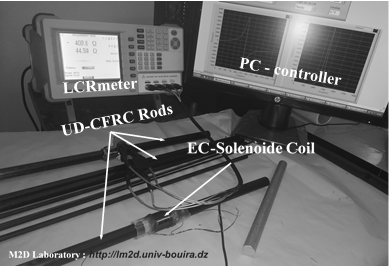Figure 1. Experimental setup performed with a precision LCRmeter

Table 1. Dimensions and electrical parameters of CFRC rod and coil 2

 UD-CFRC rod Height 300 mm UD-CFRC rod diameter 15.9 mm Height of coil 31.7 mm Coil inner diameter 16.5 mm Coil external diameter 21.7 mm Copper wire diameter 0.55 mm Coil turn number 138 Coil layer number 3 UD-CFRC rod transverse conductivity st 81 S/m Copper conductivity 58 MS/m

## 2.png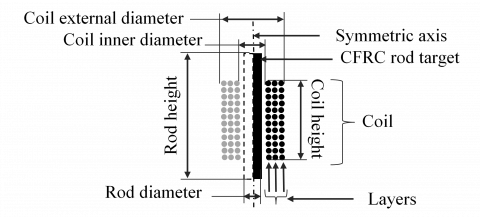Figure 2. Geometric definition of EC-NDE of CFRC rod

2.2 Global computation procedure

To illustrate the high frequency behavior of air coils, the measuring of real and imaginary impedance parts according to frequency up to 5MHz is done using a precision LCRmeter.

As appears in Figure 3, no resonance point is observed for coil 1 up to the maximum frequency, but for the coil 2 a first resonant point is detected at 1.2MHz. This is due to the fact that the number of turns in coil 2 is higher compared to the coil 1. Therefore, the equivalent capacitor of the coil 2 is more important than the coil 1 one.

The capacitive effect inside the coil 1 could be neglected when the effect of equivalent inner capacitor of UD-CFRC rod is ignored. This capacitor is outcome from the displacement currents between the fibers through the polymer matrix. Authors [8-10] proposed the equivalent electric circuit of the laminated UD-CFRC materials applied to the contact volt-ampere-meter conductivity measurement. Figure 4 shows the electrical diagram (Figure 4a) inside a UD-CFRP and equivalent circuit proposed by Jones et al. . There is only the conduction current type in the parallel direction of the fibers that expressed by an inductance in series of a resistance. But, the two current types (displacement and conduction) arise in the transversal direction. The conduction current is due to the contacts of the adjacent fibers modeled by a resistance. The displacement current appears for a high frequency, is due to the dielectric nature of the media between fibers, it is modeled by a capacitor.

The impedance variation of the coil is computed by the difference between the impedances with and without presence of the UD-CFRC rod. During the EC-NDC of these materials the impedance variation of the coil 1 is used to evaluate transversal electrical conductivity .

Due to the important number of carbon fibers impregnated in the rod and their size compared to global dimensions, the UD-CFRC rod is replaced in the FE axisymmetric model by the homogenized one with an equivalent tensor conductivity according [4-6].

## 3.png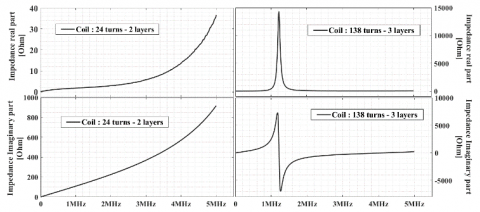Figure 3. Measured of real and imaginary part of EC-probe impedances without presence of CFRC rod target

## 4.pngFigure 4. Electrical diagram inside a UD-CFRP a) Simplified electrical diagram of UD-CFRP, b) Possible equivalent electrical circuit of UD-CFRP

FE eddy current model coupled to RLC equivalent circuit can be used to evaluate the capacitors of the coil and the UD-CFRC rod inner capacitor. This evaluation requires the multi-frequency analysis. The inversion of the model takes a lot of time, due to the important number of iterations. To reduce the computation time, a quick procedure given by the successive steps below is proposed.

1. Computing impedance of coil 2 according to frequency, using a FE axisymmetric eddy current model coupled to RL equivalent circuit without CFRC-rod.
2. Introducing the obtained impedance into the RLC equivalent circuit (Figure 5).
3. Inverse problem algorithm (Figure 6) is applied to evaluate the equivalent inter-turn capacitor C1, C2, C3, and inter-layer C12 and C23 with considering the dielectric dissipation resistances (RC1, RC2, RC3, RC12, RC23). The goal function is minimized using batt algorithm optimization (BAO).
4. The homogenized UD-CFRC rod with equivalent tensor conductivity evaluated using coil 1 as , is introduced into the FE axisymmetric model coupled to RLC equivalent circuit.

## 5.png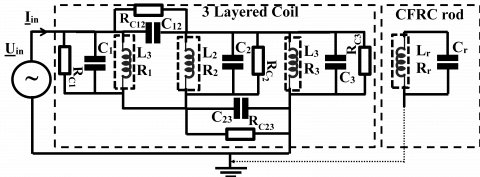Figure 5. Air-coil equivalent circuit with and without UD-CFRC rod

## 6.pngFigure 6. Inverse problem algorithm to compute

The complete equivalent circuit model can be represented as a transformer model, with the air-coil in the primary and the homogenized UD-CFRC rod in the secondary short-circuited to capacitor in parallel to resistance.

The equivalent inner capacitor Cr of the UD-CFRC rod can be obtained manually using the FE axisymmetric model coupled to RLC equivalent circuit, by the verification of the experimental first resonant point of the coil.

All evaluated parameters are introduced into the FE eddy current model coupled to the equivalent RLC circuit, to calculate impedances as a function of frequency. Then, they are compared with the measured results.

The FE models coupled to equivalent circuits and the inversion algorithm are implemented in both GNU software, FEMM  and Octave .

3. Modeling Approach

3.1 FE axisymmetric model coupled to RL equivalent circuit

Figure 2 show the geometry of the experimental setup to be modeled. The FE axisymmetric eddy current model coupled to RL equivalent circuit is expressed using magnetic vector and electrical scalar potentials Av. The FE formulation in the sub regions ke of the coil turn with the same imposed voltage $U_{k_{e}}^{\prime}$ can be written [14-16] as bellow:

$\begin{array}{c} \frac{\partial}{\partial r}\left(v^{\prime} \frac{\partial A^{\prime}}{\partial r}\right)-\frac{\partial}{\partial z}\left(v^{\prime} \frac{\partial A^{\prime}}{\partial z}\right)-j \omega \sigma_{c o}^{\prime} A^{\prime}+\sigma_{c o}^{\prime} \frac{U_{k_{e}}^{\prime}}{2 \pi} \\ =0 \end{array}$    (1)

\begin{aligned} U_{k_{e}}^{\prime}=-2 \pi r \cdot \operatorname{grad} & V_{k_{e}} \\ &=R_{k_{e}}^{\prime} I_{k_{e}}+j \omega R_{k_{e}}^{\prime} \int_{S_{k_{e}}}^{} \sigma_{c o}^{\prime} A^{\prime} d s \end{aligned}    (2)

$R_{k_{e}}^{\prime}=2 \pi / \int_{s_{k_{e}}}^{} \sigma_{c o}^{\prime} d s$    (3)

All coil turns through which the total current I flows, are in series. The current Ik in each turn kc of the coil layer kl according to the currents $I_{k_{e}}$ in the conductor sub region, is given by:

$I_{k_{c}}=\sum_{k_{e}=1}^{N_{e k c}} I_{k_{e}}=I_{k_{l}}=I$   (4)

The coil applied voltage according to the layer voltage $U_{k_{l}}$ can be expressed as:

$U_{i n}=\sum_{k_{l}=1}^{N_{L}} U_{k_{l}}$     (5)

with,

$U_{k_{l}}=\sum_{k_{c}=1}^{N_{c}} U_{k_{c}}$     (6)

where,

A': Modified magnetic vector potential (T·m3), A'=(0,$r A_{\varphi}$,0);

$\sigma_{c o}^{\prime}=\frac{\sigma_{c o}}{r}$ with σco is the copper conductivity [S/m], σco=57MS/m;

ω: pulsation of current I (rad/s); ω=$2 \pi f$;

f: is the working frequency;

ν'=$\frac{1}{r \mu_{0}}$ with μ0 is the Magnetic permeability of air,

μ0=4$\pi$10-7 H/m;

$R_{k_{e}}{ }^{\prime}$: DC resistance of the conductor sub region;

$I_{k_{l}} \text { and } U_{k_{l}}$: Electrical current and voltage on the layer $k_{l}$;

$I_{1}=I_{2}=I_{3}=\ldots=I_{k_{c}}=I_{k_{l}}=I$;

Nc: Turn number in each layer;

NL: Layer number of the coil.

The formulation in air domain, is given as follow:

$\iint_{\Omega_{a}}^{} \frac{\partial}{\partial r}\left(v^{\prime} \frac{\partial A^{\prime}}{\partial r}\right)-\frac{\partial}{\partial z}\left(v^{\prime} \frac{\partial A^{\prime}}{\partial z}\right)=0$    (7)

3.2 RLC equivalent circuit

The proposed equivalent electrical circuit of the three layers of coil without presence of the UD-CFRC rod is given by Figure 5. Where, the inductances L1, L2, L3 and the resistances R1, R2, R3 of the layer i are given by the FE axisymmetric eddy current model coupled to RL equivalent circuit as a real and imaginary parts of the layer impedance that computed as:

$R_{k_{l}}=r e\left(\frac{U_{k_{l}}}{I_{k_{l}}}\right) L_{k_{l}}=\frac{\operatorname{Im}\left(\frac{U_{k_{l}}}{I_{k_{l}}}\right)}{2 \pi f} k_{l}=1,2,3$    (8)

The equivalent impedance $Z_{e k_{l}}$ of the layer $k_{l}$ with considering the equivalent capacitor $C_{k_{l}}\left(Z_{C k_{l}}=\frac{R_{C k_{l}}}{1+j C_{k_{l}} \omega R_{C k_{l}}}\right)$ of layer i, is expressed as:

$Z_{e k_{l}}=\frac{Z_{k_{l}} Z_{C k_{l}}}{Z_{k_{l}}+Z_{C k_{l}}} k_{l}=1,2,3, \ldots, N_{L}$     (9)

The total impedance Zcal of the three layers considering the equivalent capacitors C12, C23 (Figure 5) between layers is expressed, by using the delta/star transformation , as:

$\begin{array}{c} Z_{c a l}=\frac{Z_{e 1} Z_{C 12}}{Z_{e 1}+Z_{e 2}+Z_{C 12}}+\cdots \\ \frac{\left(\frac{Z_{e 2} Z_{C 12}}{Z_{e 1}+Z_{e 2}+Z_{C 12}}+Z_{e 3}\right)\left(\frac{Z_{e 1} Z_{e 2}}{Z_{e 1}+Z_{e 2}+Z_{C 12}}+Z_{C 23}\right)}{\frac{Z_{e 2} Z_{C 12}+Z_{e 1} Z_{e 2}}{Z_{e 1}+Z_{e 2}+Z_{C 12}}+Z_{C 23}+Z_{e 3}} \end{array}$   (10)

where, ZC12 and ZC23 are the equivalent impedances of C12 and C23 with considering the dielectric dissipation resistances.

All capacitors of the coil are evaluated using the inverse problem algorithm illustrated in Figure 6. The difference between the measured and computed impedances, expressed by the goal function FG, is minimized until verification of the convergence criterion. The inverse problem goal function FG is written as:

$F_{G}=\sqrt{\frac{1}{N_{f}} \sum_{k=1}^{N_{f}} \frac{1}{2}\left[\left(\frac{R_{m_{k}}-R_{c_{k}}}{R_{m_{k}}}\right)^{2}+\left(\frac{X_{m e s_{k}}-X_{c_{k}}}{X_{m_{k}}}\right)^{2}\right]}$    (11)

where, Rmk and Xmk  are successively real and imaginary parts of the measured impedance at the frequency point k.

Rck and Xck are successively real and imaginary parts of the computed impedance at the frequency point k.

Nf: is the number of measured points.

The inner capacitor of the UD-CFRC rod will be evaluated manually using the FE axisymmetric model coupled to RLC equivalent circuit given in next sub section.

Bat algorithm optimization  implemented in Octave® is used to minimizing the goal function.

3.3 FE axisymmetric model coupled to RLC equivalent circuit

To take into account the capacitive effect in the UD-CFRC, it will be modeled by a solid conductor in parallel with a capacitor and a dielectric losses resistor. The coil layers are also replaced by the solid conductors in parallel to capacitors and resistors.

The complete equivalent circuit model can be represented as a transformer model.

Eqns. (1) and (2) that written in the sub domain turn coil remain unchanged. Eq. (4) becomes:

$I_{k_{c}}=I_{k_{l}}=\sum_{k_{e}=1}^{N_{e k c}} I_{k_{e}}=\frac{U_{k_{l}}}{Z_{k_{l}}}$    (12)

The total current I, is obtained by dividing the input voltage by the computed impedance given by Eq. (9):

$I=\frac{U_{i n}}{Z_{c a l}}$    (13)

Eq. (6) remains unchanged but (5) is rewritten by several equation using meshes law of Kirchhoff applied to the electrical equivalent circuit illustrated in Figure 5.

To write the FE formulation in the homogenized UD-CFRC rod target we replace $\sigma_{C O}^{\prime}$ will be replaced by $\sigma_{t}^{\prime}$ in Eq. (1). $\sigma_{t}^{\prime}=\frac{\sigma_{t}}{r}$ with σt is a transverse conductivity of the UD-CFRC rod [S/m];

Eq. (2) is also written for the UD-CFRC by replacing the conductivity as above.

The equivalent inner capacitor Cr of the UD-CFRC rod is be obtained manually using the presented FE axisymmetric model coupled to RLC equivalent circuit, by the verification of the experimental first resonant point of the coil.

4. Results and Discussion

Figure 7 shows both the real and imaginary parts of the coil impedance measured with the LCRmeter and computed using the classical model where the capacitive effect is neglected. The both cases, coil only and coil with UD-CFRC rod are considered.

## 7-1.png## 7-2.png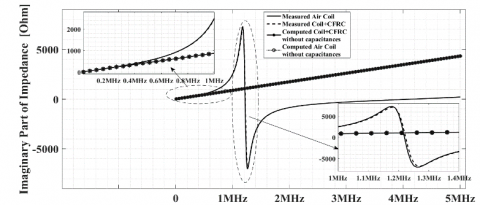Figure 7. Computed and measured impedance without considering capacitors

We can see that the classical model diverges after the frequency of 0.5MHz. Consequently, from this frequency the capacitive effect is very significant for the considered coil. Thereby, the classical model is not convenient for the frequency greater than 0.5MHz. The proposed approach in this article, allows this effect to be taken into account for all frequencies.

On the other hand, the measurements show that the presence of the UD-CFRC rod affects the resonant point of the coil. Where, the resonance frequency increases and the amplitude of the impedance decreases. For the considered coil, the frequency at the resonant point for coil only is 1219 kHz and become 1223 kHz with presence of UD-CFRC rod. At the resonant point, the amplitude of the impedance for coil only is 14.5 kW and become 13.8 kW with presence of UD-CFRC rod.

These observed phenomena are exploited to evaluate the equivalent inner capacitor Crof the UD-CFRC rod, using the proposed model.

Table 2 show the equivalent capacitors evaluated using the procedure that illustrated in subsection 2.2.

After introducing all evaluated equivalent capacitors to the FE axisymmetric model coupled to RLC equivalent circuit with the presence of the UD-CFRC rod, the impedance is computed for several frequencies up to 5MHz and then it is compared to the measured ones (Figure 8). We can see that the computed impedances obtained with proposed model follow exactly the measured ones. A mean error less than 2% and a maximum one of 5% are observed.

Table 2. Identified parameters of the model

 C1 C2 C3 C12 C23 Cr 55pF 65pF 75pF 100pF 120pF 50uF RC1 RC2 RC3 RC12 RC23 RC23 21.4$\mathrm{k} \Omega$ 21.4$\mathrm{k} \Omega$ 21.4$\mathrm{k} \Omega$ 50$\mathrm{k} \Omega$ 50$\mathrm{k} \Omega$ $\infty$

## 8-1.png## 8-2.pngFigure 8. Computed and measured impedance with considering capacitive

5. Conclusion

In order to taking into account of the capacitive effect in eddy current NDC/NDT model of UD-CFRC rod, a new fast procedure is proposed. The inter-spire and inter-layer equivalent capacitors of the air coil are firstly evaluated using the RLC equivalent circuit and the FE axisymmetric model coupled to RL equivalent circuit. Then the FE axisymmetric model coupled to RLC equivalent circuit is applied to identify the inner capacitor of the UC-CRFC, by adjusting the resonant point.

To show the effectiveness of the proposed approach, the computed results are confronted to the measured ones, with and without considering capacitive effect. The comparison between the experimental and simulation results, shows a good concordance with a mean error less than 2% according to frequency.

The proposed approach can also be used in the case of the stratified CFRC plate, but the 3D model is required.

Nomenclature
 A magnetic vector potential, T.m-2 C capacitance, F f frequency, Hertz I electrical current, A r radial coordinate, m R electrical resistance, Ohm U voltage, V v electrical potential, V X reactance, Ohm Z electrical impedance, Ohm Greek symbols $\sigma_{t}$ transverse conductivity of the UD-CFRC rod, S/m µ magnetic permeability, H/m Subscripts $Z_{e k_{l}}$ equivalent impedance of the coil layer kl FG objective function or goal function
References

 Bakis, C.E., Bank, L.C., Brown, V., Cosenza, E., Davalos, J.F., Lesko, J.J., Triantafillou, T.C. (2002). Fiber-reinforced polymer composites for construction—State-of-the-art review. Journal of Composites for Construction, 6(2): 73-87. https://doi.org/10.1061/(ASCE)1090-0268(2002)6:2(73)

 Basim, S., Hejazi, F., Rashid, R.S.B.M. (2019). Embedded carbon fiber-reinforced polymer rod in reinforced concrete frame and ultra-high-performance concrete frame joints. International Journal of Advanced Structural Engineering, 11(1): 35-51. https://doi.org/10.1007/s40091-019-00253-7

 Koyama, K., Hoshikawa, H., Kojima, G. (2013). Eddy current nondestructive testing for carbon fiber-reinforced composites. Journal of Pressure Vessel Technology, 135(4): 041501. https://doi.org/10.1115/1.4023253

 Bensaid, S., Trichet, D., Fouladgar, J. (2009). Electrical conductivity identification of composite materials using a 3-D anisotropic shell element model. IEEE Transactions on Magnetics, 45(3): 1859-1862. https://doi.org/10.1109/TMAG.2009.2012833

 Bensaid, S., Trichet, D., Fouladgar, J. (2015). Optimal design of a rotating eddy-current probe—Application to characterization of anisotropic conductive materials. IEEE Transactions on Magnetics, 51(3): 1-4. https://doi.org/10.1109/TMAG.2014.2363174

 Safer, O.A., Bensaid, S., Trichet, D., Wasselynck, G. (2017). Transverse electrical resistivity evaluation of rod unidirectional carbon fiber-reinforced composite using eddy current method. IEEE Transactions on Magnetics, 54(3): 1-4. https://doi.org/10.1109/TMAG.2017.2751962

 Wasselynck, G., Trichet, D., Ramdane, B., Fouladgar, J. (2010). Microscopic and macroscopic electromagnetic and thermal modeling of carbon fiber reinforced polymer composites. IEEE Transactions on Magnetics, 47(5): 1114-1117. https://doi.org/10.1109/TMAG.2010.2073456

 Wasselynck, G., Trichet, D., Fouladgar, J. (2013). Determination of the electrical conductivity tensor of a CFRP composite using a 3-D percolation model. IEEE Transactions on Magnetics, 49(5): 1825-1828. https://doi.org/10.1109/TMAG.2013.2241039

 Bui, H.K., Senghor, F. D., Wasselynck, G., Trichet, D., Fouladgar, J., Lee, K., Berthiau, G. (2017). Characterization of electrical conductivity of anisotropic CFRP materials by means of induction thermography technique. IEEE Transactions on Magnetics, 54(3): 1-4. https://doi.org/10.1109/TMAG.2017.2742979

 Piche, A., Revel, I., Peres, G., Pons, F., Lazorthes, B., Gauthier, B., Cadaux, P.H. (2009). Numerical modeling support for the understanding of current distribution in carbon fibers composites. In 2009 International Symposium on Electromagnetic Compatibility-EMC Europe, pp. 1-4. https://doi.org/10.1109/EMCEUROPE.2009.5189695

 Jones, C.E., Norman, P.J., Galloway, S.J., Burt, G.M., Kawashita, L.F., Jones, M.I., Hallett, S.R. (2016). Electrical model of carbon fibre reinforced polymers for the development of electrical protection systems for more-electric aircraft. In 2016 18th European Conference on Power Electronics and Applications (EPE'16 ECCE Europe), pp. 1-10. https://doi.org/10.1109/EPE.2016.7695300

 Meeker, D. (2010). Finite element method magnetics. FEMM, 4, 32. https://www.femm.info/.

 Eaton, J.W., Bateman, D., Hauberg, S. (1997). Gnu octave (p. 42). London: Network theory. https://www.gnu.org/software/octave/

 Bastos, J.P.A., Sadowski, N. (2003). Electromagnetic Modeling by Finite Element Methods. CRC press.

 Lombard, P., Meunier, G. (1993). A general purpose method for electric and magnetic combined problems for 2D, axisymmetric and transient systems. IEEE Transactions on Magnetics, 29(2): 1737-1740. https://doi.org/10.1109/20.250741

 Bensaid, S. (2006). Contribution à la caractérisation et à la modélisation électromagnétique et thermique des matériaux composites anisotropes. Ph.D. Dissertation, Nantes University.

 Kennelly, A.E. (1899). The equivalence of triangles and three-pointed stars in conducting networks. Electrical World and Engineer, 34(12): 413-414.

 Yang, X.S., Gandomi, A.H. (2012). Bat algorithm: a novel approach for global engineering optimization. Engineering Computations, 29(5): 464-483. https://doi.org/10.1108/02644401211235834